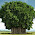## Wednesday, March 17, 2010

### Excel Formula : Subtotal Excel

Most of the time in our reporting we need to do subtotals followed by Grand total at the end of report. I have seen many people using SUM function for subtotals in between and then adding individual cells for calculating grand total at the end. There is nothing wrong with this method if you use it very carefully so that you do not miss any item while calculating grand total at the end.

However better approach is to use Excel Subtotal function for calculating Subtotal and Grand Totals.Image above is showing column B with the Subtotal formula and resultant values with this formula. You may notice that when we use subtotal function for calculating Grand Total, it ignores the values resulting from subtotal function within its range. This method ensures that we do not miss any item while doing Grand Totals for our reports thus higher accuracy.

This becomes more helpful when you edit your report after some time to insert another subtotal. You do not need to remember to correct the final formula calculating the Grand Total.

Another advantage of using Subtotal function is that you need not to recalculate your formulas when used with filteres. This function ignores the values hidden by the filters and considers the values in the visible rows only.

Subtotal can be used for various type of calculations based on what do you want this fucntion to do for you. Syntex for this function is

SUBTOTAL(function_num, ref1, ref2, ...)

Following function numbers can be used in Subtotal function

I have used "9" in my example above for sum you can change it as per your required working.

Function_num
(Incl hidden values)
Function_num
(Excl hidden values)
Function
1101AVERAGE
2102COUNT
3103COUNTA
4104MAX
5105MIN
6106PRODUCT
7107STDEV
8108STDEVP
9109SUM
10110VAR
11111VARP

1.sorry, it shows error

2.Hi Seema

Can you please let me know what is the formula you have used. Just cut and paste your formula. Also let me know which version of excel you are using.

Regards
Yogesh Gupta

3.Dear Yogesh do u have a solution for the password protected excel sheet...how can we view the same

4.Hi Izhaar

I do not have a solution for this.

Thanks

5.hi Yogesh

how to calculate average of 24 hours time like 23:25,22:10,00:20

Regards,

Gautam

6.hi sir..
Im vishal patel.

I want to daily interest calculator.
Means. If im give 1 lacs rs. for interest to any person. rate of interest 1% per month.
After i give other 1.5 lacs after 15 days so, i want to total count interest for 1 lacs for 30 days (1 moth) and other 1.5 lack interest (15 Days) How much it?

call 9998011141

7.This comment has been removed by the author.

8.Hello Sir..
I need formula for my records...I have three columns of data...in which I have to subtract 2 columns..then I have to divide with the subtracted answer..but the #div0 error comes ...please guide me how can I use IF or ISBLANK Function.

B2= 40,101...
B3= Blank[sometime]
B4= Blank[sometime]

=(B2-B3)/B4

This 3 columns in initial stage remaining blank..After few days the data starts to fulfill.

Thanks,
Dipsdoshi

October 22, 2012 5:28 PM

9.hai sir how are you? my name is Raja, I am working in Vlookup function, i want continues printout in the invoices Eg:1 to 5 how to use please help me sir, my email id skraja.2009@gmail.com

10.11.dear sir,
i need help for following, i want result in col c4 , - 1:00 hrs pls advise.
1 A B C

2 DUTY REG OT

3 12:30 10:00 2:30
4 9:00 10:00 =A4-B4 (result
showing ####